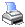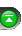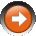﻿ Branching Commands
 NinjaScript > Educational Resources > Basic Programming Concepts > Branching CommandsBranching controls execution flow of your script. It allows you to branch into separate logical sequences based on decisions you make.

The if Statement

An if statement allows you to take execute different paths of logic depending on a given condition.

 // Single case condition int x = 0; if (x == 0) {     Print("NinjaTrader"); }

The above example will print NinjaTrader to the NinjaTrader output window since x does equal 0.

 // Either/Or decision int x = 1; if (x == 0) {     Print("NinjaTrader"); } else {     Print("NinjaScript"); }

The above example will print NinjaScript to the NinjaTrader output window.

 // Multiple case decision int x = 2; if (x == 0) {     Print("NinjaTrader"); } else if (x == 1) {     Print("NinjaScript"); } else {     Print("NinjaTrader Rules!"); }

The above example will print NinjaTrader Rules! to the NinjaTrader output window.

The switch Statement

The switch statement executes a set of logic depending on the value of a given parameter.

 // Switch example int x = 2; switch (x) {     case 0:          Print("x is equal to zero");         break;     case 1:          Print("x is equal to one");         break;     case 2:          Print("x is equal to two");         break; }

The above example will print out x is equal to two to the NinjaTrader output window.